Home | | Physics 11th std | Long Questions and Answer

## Chapter: 11th Physics : UNIT 11 : Waves

Physics : Waves : Book Back Important Questions, Answers, Solutions : Long Questions and Answer

Waves (Physics)

1. Discuss how ripples are formed in still water.

● A stone is dropped in a trough of still water, we can see a disturbance produced at the place where the stone strikes the water surface. This disturbance spreads out ( diverges out) in the form of concentric circles of ever increasing radii (ripples) and strike the boundary of the trough.

● This is because some of the kinetic energy of the stone is transmitted to the water molecules on the surface. Actually the particles of the water (medium) themselves do not move outward with the disturbance.

● This can be observed by keeping a paper strip on the water surface. The strip moves up and down when the disturbance (wave) passes on the water surface. This shows that the water molecules only undergo vibratory motion about their mean positions.

2. Briefly explain the difference between travelling waves and standing waves.Progressive waves

1. Crests and troughs are formed in transverse progressive waves, and compression and rarefaction are formed in longitudinal progressive waves.

2. These waves move forward or backward in a medium i.e., they will advance in a medium with a definite velocity.

3. All the particles in the medium vibrate such that the amplitude of the vibration for all particles is same.

4. These wave carry energy while propagating.

Stationary waves

1. Crests and troughs are formed in transverse stationary waves, and compression and rarefaction are formed in longitudinal stationary waves.

2. These waves neither move forward nor backward in a medium i.e., they will not advance in a medium.

3. Except at nodes, all other particles of the medium vibrate such that amplitude of vibration is different for different particles. The amplitude is minimum or zero at nodes and maximum at anti-nodes.

4. These waves do not transport energy.

3. Show that the velocity of a travelling wave produced in a string is v = √[T/μ]

● Consider an elemental segment in the string as shown in the Figure. Let A and B be two points on the string at an instant of time.

● Let dl and dm be the length and mass of the elemental string, respectively.

By definition, linear mass density, μ is μ = dm/dl …..(1)

dm = μ dl ………….. (2)● The elemental string AB has a curvature which looks like an arc of a circle with centre at O, radius R and the arc subtending an angle θ at the origin O.

● The angle θ can be written in terms of arc length and radius as θ = dl / R. The centripetal acceleration supplied by the tension in the string is a acp = v2 / R  ………..(3)

● Then, centripetal force can be obtained when mass of the string (dm) is included in equation (3)

Fcp = (dm) v2 / R     ………..(4)

● The centripetal force experienced by elemental string can be calculated by substituting equation (2) in equation (4) we get

(dm)v2 / R = μv2dl / R    ……. (5)

● The tension T acts along the tangent of the elemental segment of the string at A and B. Since the arc length is very small, variation in the tension force can be ignored. We can resolve T into horizontal component T cos (θ / 2) and vertical component T sin (θ / 2).

● The horizontal components at A and B are equal in magnitude but opposite in direction; therefore, they cancel each other.

● Since the elemental arc length AB is taken to be very small, the vertical components at A and B appears to acts vertical towards the centre of the arc and hence, they add up. The net radial force, Fr = 2T sin (θ / 2)         ……. (6)

● Since the amplitude of the wave is very small when it is compared with the length of the string, the sine of small angle is approximated as sin (θ / 2) (θ / 2).

● Hence, equation (6) can be written as Fr = 2T × (θ/ 2) = T θ  ……. (7)

● But θ = dl / R, therefore substituting in equation (7), we get Fr = T (dl/R)       …………. (8)

● Applying Newton's second law to the elemental string in the radial direction, under equilibrium, the radial component of the force is equal to the centripetal force.

● Hence equating equation (5) and equation (8), we have………… (9)

4. Describe Newton’s formula for velocity of sound waves in air and also discuss the Laplace’s correction.

● Newton assumed that when sound propagates in air, the formation of compression and rarefaction takes place in a very slow manner so that the process is isothermal in nature.

● The heat produced during compression (pressure increases, volume decreases), and heat lost during rarefaction (pressure decreases, volume increases) occur over a period of time such that the temperature of the medium remains constant.

● Therefore, by treating the air molecules to form an ideal gas, the change in pressure and volume obey Boyle's law,

PV = Constant          ………… (1)

● Differentiating equation (1), we get PdV + VdP = 0 or P = − V (dP/dV) = BT        ………… (2)

where, BT is an isothermal bulk modulus of air. Substituting equation (2) in equation v = √(B/ρ) the speed of sound in air is vT = √(Br/ρ) = √(P/ρ) ………… (3)

● Since P is the pressure of air whose value at NTP (Normal Temperature and Pressure) is 76 cm of mercury, we have

P = (0.76 × 13.6 × 103 × 9.8) N m−2

ρ = 1.293 kg m−3  here ρ is density of air

● Then the speed of sound in air at Normal Temperature and Pressure (NTP) is

vT= 279.80 ms−1 ≈ 280 ms−1  (theoretical value)

● But the speed of sound in air at 0oC is experimentally observed as 332 ms−1 which is close upto 16% more than theoretical value (Percentage error is [ (332 – 280) / 332 ] × 100% = 15.6%) This error is not small.

Laplace's correction:

● Laplace assumed that when the sound propagates through a medium, the particles oscillate very rapidly such that the compression and rarefaction occur very fast.

● The exchange of heat produced due to compression and cooling effect due to rarefaction do not take place, because, air (medium) is a bad conductor of heat.

● Since, temperature is no longer considered as a constant here, sound propagation is an adiabatic process.

● By adiabatic considerations, the gas obeys Poisson's law (not Boyle's law as Newton assumed), which is PVγ = Constant      ………… (4)

● Where, γ = CP / CV , which is the ratio between specific heat at constant pressure and specific heat at constant volume. Differentiating equation (4) on both the sides, we get Vγ dP + P (γVγ −1 dV) = 0 (or) γP = −V (dP / dV) = BA

where, BA is the adiabatic bulk modulus of air. Now, substituting equation (5) in equation v = √(B/ρ) the speed of sound in air is5. Write short notes on reflection of sound waves from plane and curved surfaces.● Sound also reflects from a harder flat surface, This is called as specular reflection.

● Specular reflection is observed only when the wavelength of the source is smaller than dimensions of the reflecting surface, as well as smaller than surface irregularities.

● When the sound waves hit the plane wall, they bounce off in a manner similar to that of light. Suppose a loudspeaker is kept at an angle with respect to a wall (plane surface), then the waves coming from the source (assumed to be a point source) can be treated as spherical wave fronts.

● Therefore, the reflected wave front on the plane surface is also spherical, such that its centre of curvature can be treated as the image of the sound source (virtual or imaginary loud speaker) which can be assumed to be at a position behind the plane surface.

Reflection of sound through the curved surface:

● The behaviour of sound is different when it is reflected from different surfaces-convex or concave or plane.

● The sound reflected from a convex surface is spread out and so it is easily attenuated and weakened.

● Whereas, if it is reflected from the concave surface it will converge at a point and this can be easily amplified.

● The parabolic reflector (curved reflector) which is used to focus the sound precisely to a point is used in designing the parabolic mics which are known as high directional microphones.

● Any surface either smooth or rough can absorb sound.

● For example, the sound produced in a big hall or auditorium or theatre is absorbed by the walls, ceilings, floor, seats etc.

6. Briefly explain the concept of superposition principle.

● When a jerk is given to a stretched string which is tied at one end, a wave pulse is produced and the pulse travels along the string.

● Suppose two persons holding the stretched string on either side give a jerk simultaneously, then these two wave pulses move towards each other, meet at some point and move away from each other with their original identity.

● Their behaviour is very different only at the crossing/ meeting points; this behaviour depends on whether the two pulses have the same or different shape as shown in Figure.● When the pulses have the same shape, at the crossing, the total displacement is the algebraic sum of their individual displacements and hence its net amplitude is higher than the amplitudes of the individual pulses.

● If the two pulses have same amplitude but shapes are 180° out of phase at the crossing point, the net amplitude vanishes at that point and the pulses will recover their identities after crossing.

● Only waves can possess such a peculiar property and it is called superposition of waves. This means that the principle of superposition explains the net behaviour of the waves when they overlap.

● Generalizing to any number of waves i.e, if two are more waves in a medium move simultaneously, when they overlap, their total displacement is the vector sum of the individual displacements.

● To understand mathematically, let us consider two functions which characterize the displacement of the waves, for example, y1 = A1 sin (kx - ωt) and y2 = A2 cos(kx - ωt)

● Since, both y1 and y2 satisfy the wave equation (solutions of wave equation) then their algebraic sum y = y1 + y2 also satisfies the wave equation.

● This means, the displacements are additive. Suppose we multiply y1 and y2 with some constant then their amplitude is scaled by that constant. Further, if C1 and C2 are used to multiply the displacements y1 and y2, respectively, then, their net displacement y is y = C1y1 + C2y2

● This can be generalized to any number of waves. In the case of n such waves in more than one dimension the displacements are written using vector notation.

Here, the net displacement● The principle of superposition can explain the following:

(a) Space (or spatial) Interference (also known as Interference)

(b) Time (or Temporal) Interference (also known as Beats)

(c) Concept of stationary waves

● Waves that obey principle of superposition are called linear waves

● If the amplitude of the wave is not small then they are called non-linear waves.

7. Explain how the interference of waves is formed.

● Consider two harmonic waves having identical frequencies, constant phase difference φ and same wave form (can be treated as coherent source), but having amplitudes A1 and A2, then

y1 = A1 sin(kx − ωt) ………. (1)

y2 = A2 sin(kx − ωt + φ) ………… (2)

● Suppose they move simultaneously in a particular direction, then interference occurs

(i.e., overlap of these two waves), y = y1 + y2      ……… (3)

● Therefore, substituting equation (1) and equation (2) in equation (3), we get

y = A1 sin(kx − ωt) + A2 sin(kx − ωt + φ)

Using trigonometric identity sin (α + β) = (sinα cosβ + cos α sinβ), we get

● y = A1 sin(kx − ωt)+A2 [sin(kx − ωt) cosφ + cos(kx − ωt) sinφ]

● y = sin(kx − ωt)( A1 + A2 cosφ) + A2 sinφ cos(kx − ωt)      ……… (4)

A cosθ = ( A1 + A2 cosφ) ……….. (5)

and A sinθ = A2 sinφ ………….. (6)

● then equation (4) can be rewritten as

y = A sin(kx − ωt) cosθ + A cos(kx − ωt) sinθ

y = A (sin (kx − ωt) cosθ + sinθ cos(kx − ωt))

y = A sin(kx – ωt + θ) …………… (7)

● By squaring and adding equation (5) and equation (6), we get

A2 = A12 + A22 + 2A1A2 cosφ ………… (8)

● Since, intensity is square of the amplitude (I = A2),

● we have I = I1 + I2 + 2√(I1 I2)cosφ  ……….. (9)

● This means the resultant intensity at any point depends on the phase difference at that point.

(a) For constructive interference:

● When crests of one wave overlap with crests of another wave, their amplitudes will add up and we get constructive interference.

● The resultant wave has a larger amplitude than the individual waves.● The constructive interference at a point occurs if there is maximum intensity at that point, which means that cosφ = + 1 => φ = 0, 2π, 4π, ... = 2n π, where n = 0,1,2,...

● This is the phase difference in which two waves overlap to give constructive interference. Therefore, for this resultant wave,

Imaxhnum = (√I1 + √I2)2 = ( A1 + A2)2

● Hence, the resultant amplitude A = A1 + A2

(b) For destructive interference:

● When the trough of one wave overlaps with the crest of another wave, their amplitudes "cancel" each other and we get destructive interference. The resultant amplitude is nearly zero.

● The destructive interference occurs if there is minimum intensity at that point, which means cosφ = − 1 => φ = π, 3 π, 5 π,... = 2(n − 1) π,

where n = 0,1,2,.... i.e. This is the phase difference in which two waves overlap to give destructive interference.

Therefore, Iminimum = (√I1 − √I2)2 = ( A1 − A2)2

● Hence, the resultant amplitude A= | A1 − A2 |

8. Describe the formation of beats.

Formation of beats:

● When two or more waves superimpose each other with slightly different frequencies, then a sound of periodically varying amplitude at a point is observed. This phenomenon is known as beats. The number of amplitude maxima per second is called beat frequency.

● If we have two sources, then their difference in frequency gives the beat frequency.

● Number of beats per second n = | f1 f2 | Per second

9. What are stationary waves?. Explain the formation of stationary waves and also write down the characteristics of stationary waves.

● When the wave hits the rigid boundary it bounces back to the original medium and can interfere with the original waves.

● A pattern is formed, which are known as standing waves or stationary waves.

● Consider two harmonic progressive waves (formed by strings) that have the same amplitude and same velocity but move in opposite directions. Then the displacement of the first wave (incident wave) is

y1 = A sin(kx − ωt) (waves move toward right) …… (1)

and the displacement of the second wave (reflected wave) is

y2 = A sin(kx + ωt) (waves move toward left) …….. (2)

both will interfere with each other by the principle of superposition, the net displacement is y = y1+ y2 ……(3)

● Substituting equation (1) and equation (2) in equation (3), we get

y = A sin(kx - ωt) + A sin(kx + ωt) ……….. (4)

● Using trigonometric identity, we rewrite equation (4) as

y (x, t) = 2A cos(ωt) sin(kx) ………… (5)

● This represents a stationary wave or standing wave, which means that this wave does not move either forward or backward, whereas progressive or travelling waves will move forward or backward.

● Further, the displacement of the particle in equation (5) can be written in more compact form, y(x, t) = A' cos(ωt) where, A' = 2A sin(kx), implying that the particular element of the string executes simple harmonic motion with amplitude equals to A'.

● The maximum of this amplitude occurs at positions for which

sin(kx) =1 => kx = π/2, 3π/2, 5π/2, ….. m π

where m takes half integer or half integral values. The position of maximum amplitude is known as antinodes.

Characteristics of stationary waves :

● Stationary waves are characterized by the confinement of a wave disturbance between two rigid boundaries.

● This means, the wave does not move, forward or backward in a medium (does not advance), it remains steady at its place. Therefore, they are called "stationary waves or standing waves".

● Certain points in the region in which the wave exists have maximum amplitude, called as anti-nodes and at certain points the amplitude is minimum or zero, called as nodes.

● The distance between two consecutive nodes (or) anti-nodes is λ / 2

● The distance between a node and its neighbouring anti-node is λ / 4

● The transfer of energy along the standing wave is zero

10. Discuss the law of transverse vibrations in stretched strings.

i) The law of length:

For a given wire with tension T (which is fixed) and mass per unit length μ (fixed) the frequency varies inversely with the vibrating length.

Therefore, f 1/l => f = C/l => l × f = C, where C is a constant.

ii) The law of tension:

For a given vibrating length l (fixed) and mass per unit length μ (fixed) the frequency varies directly with the square root of the tension T, f √T

f= A√T where A is a constant

iii) The law of mass:

For a given vibrating length l (fixed) and tension T (fixed) the frequency varies inversely with the square root of the mass per unit length μ,  f 1/√μ f = B/√μ , where B is a constant.

11. Explain the concepts of fundamental frequency, harmonics and overtones in detail.

● The rigid boundaries at x = 0 and x = L and produce a standing waves by wiggling the string. Standing waves with a specific wavelength are produced.

● Since, the amplitude must vanish at the boundaries, therefore, the displacement at the boundary y(x = 0, t) = 0 and y(x = L, t) = 0 ………… (1)

Since the nodes formed are at a distance λn/2 apart, we have nn/2) = L

where n is an integer, L is the length between the two boundaries and λn is the specific wavelength

λn = (2L/n)       ……….(2)

● The (allowed) wavelengths should fit with the specified boundary conditions, i.e., for n = 1, the first mode of vibration has specific wavelength λ1 = 2L. Similarly for n = 2,

the second mode of vibration has specific wavelength λ2 = λ2 = (2L/2)= L

For n = 3, the third mode of vibration has specific wavelength λ3 = (2L/3) and so on.

● The frequency of each mode of vibration ( called natural frequency ) can be calculated.

We have, fn = v / λn = n(v / 2L)     ……….(3)

● The lowest natural frequency is called the fundamental frequency.

f1 = v / λ1 = (v / 2L)   ……….(4)

● The second natural frequency is called the first over tone.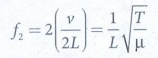● The third natural frequency is called the second over tone.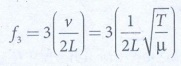and so on. Therefore, the nth natural frequency can be computed as integral (or integer) multiple of fundamental frequency, i.e.,

fn = nf1 where n is an integer  ………. (5)

● If natural frequencies are written as integral multiple of fundamental frequencies, then the frequencies are called harmonics. Thus, the first harmonic is f1 = f1 (the fundamental frequency is called first harmonic), the second harmonic is f2 = 2f1, the third harmonic is f3 = 3f1 etc.

12. What is a sonometer?. Give its construction and working. Explain how to determine the frequency of tuning fork using sonometer.

● Sono means sound related, and sonometer implies sound-related measurements.

● It is a device for demonstrating the relationship between the frequency of the sound produced in the transverse standing wave in a string, and the tension, length and mass per unit length of the string.

We can determine the following quantities:

(a) the frequency of the tuning fork or frequency of alternating current

(b) the tension in the string

(c) the unknown hanging mass

Construction:

● The sonometer is made up of a hollow box which is one meter long with a uniform metallic thin string attached to it.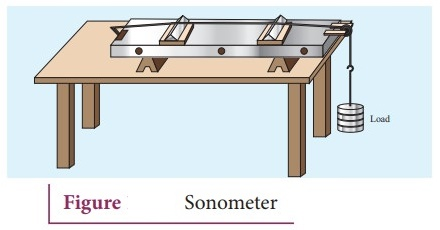● One end of the string is connected to a hook and the other end is connected to a weight hanger through a pulley.

● Since only one string is used, it is also known as monochord.

● The weights are added to the free end of the wire to increase the tension of the wire.

● Two adjustable wooden knives are put over the board, and their positions are adjusted to change the vibrating length of the stretched wire.

Working:

● A transverse stationary or standing wave is produced at the knife edges P and Q, nodes are formed. In between the knife edges, anti-nodes are formed.

● If the length of the vibrating element is l then l = λ / 2 => λ = 2 l

● Let f be the frequency of the vibrating element, T, the tension of the string and μ, the mass per unit length of the string.

● Using equation √(T/μ) we get fin Hertz.

● Let ρ be the density of the material of the string and d be the diameter of the string. Then the mass per unit, length μ,

μ = Area × density = πr2ρ = πρd2 / 4

f = v/ λ13. Write short notes on intensity and loudness.

Intensity of sound:

● When a sound wave is emitted by a source, the energy is carried to all possible surrounding points. The average sound energy emitted or transmitted per unit time or per second is called sound power.

● The intensity of sound is defined as "the sound power transmitted per unit area taken normal to the propagation of the sound wave".

● For a particular source (fixed source), the sound intensity is inversely proportional to the square of the distance from the source.

I = Power of the source / 4πr2

I 1 / r2

This is known as inverse square law of sound intensity.

Loudness of sound:

● Two sounds with same intensities need not have the same loudness. For example, the sound heard during the explosion of balloons in a silent closed room is very loud when compared to the same explosion happening in a noisy market.

● Though the intensity of the sound is the same, the loudness is not. If the intensity of sound is increased then loudness also increases.

● Loudness depends on both intensity of sound wave and sensitivity of the ear whereas the intensity of sound does not depend on the observer.

● The loudness of sound is defined as "the degree of sensation of sound produced in the ear or the perception of sound by the listener".

14. Explain how overtones are produced in a

(a) Closed organ pipe

(b) Open organ pipe

(a) Closed organ pipe:

● It is a pipe with one end closed and the other end open. If one end of a pipe is closed, the wave reflected at this closed end is 180° out of phase with the incoming wave.

● Thus there is no displacement of the particles at the closed end nodes are formed at the closed end and anti-nodes are formed at open end.

● Consider the simplest mode of vibration of the air column called the fundamental mode. Anti-node is formed at the open end and node at closed end.● Let L be the length of the tube and λ be the wavelength of the wave produced. For the fundamental mode of vibration.

L = λ1 / 4 or λ1 = 4L The frequency of the note emitted is f1= v / λ1 = v / 4L which is called the fundamental note.

● The frequencies higher than fundamental frequency can be produced by blowing air strongly at open end. Such frequencies are called overtones.

● The Figure 2 shows the second mode of vibration having two nodes and two antinodes.4L = 3 λ2 ; L = 3λ2 / 4 or λ2 = 4L / 3

The frequency for this, f2 = v / λ2 = 3v / 4L = 3 f1 is called first over tone, since here, the frequency is three times the fundamental frequency it is called third harmonic.

● The Figure 3 shows third mode of vibration having three nodes and three anti-nodes4L = 5λ3 ; L = 5λ3 / 4 or λ3 = 4L / 5 ;

The frequency f3 = v / λ3 = 5v / 4L = 5 f1 is called second over tone, and n = 5 is called fifth harmonic. The closed organ pipe has only odd harmonics and frequency of the harmonic is f n = (2n+l) f1 . Therefore, the frequencies of harmonics are in the ratio f1 : f2: f3: f4 :….. = 1 : 3 : 5 : 7 : ……

(b) Open organ pipes:

● It is a pipe with both the ends open. At both open ends, anti-nodes are formed. Let us consider the simplest mode of vibration of the air column called fundamental mode.● Since anti-nodes are formed at the open end, a node is formed at the mid-point of the pipe.

● If L be the length of the tube, the wavelength of the wave produced is given by

L = λ1 / 2 or λ1 = 2L ;

● The frequency of the note is f1 = v / λ1 = v / 2L which is called the fundamental note.● The frequencies higher than fundamental frequency can be produced by blowing air strongly at one of the open ends. Such frequencies are called overtones.

● The Figure shows the second mode of vibration in open pipes. It has two nodes and three anti-nodes, and therefore, L = λ2 or λ2 = L. The frequency f2 = v / λ2 = v / L is called first over tone. If n = 2, it is called the second harmonic.

● The Figure shows the third mode of vibration having three nodes and four anti- nodes

L = 3λ3 / 2 or λ3 = 2L / 5.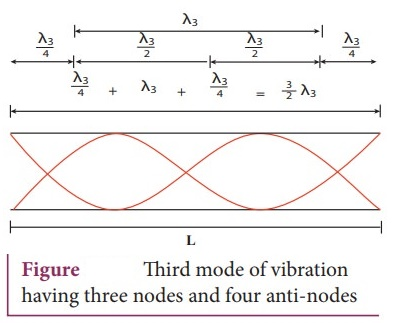The frequency f3 = v / λ3 = 3v / 2L = 3 × [ v / 2L] = 3 f1 is called second over tone. If n = 3, it is called the third harmonic.

● The open organ pipe has all the harmonics and frequency of nth harmonic is fn = n f1. Therefore, the frequencies of harmonics are in the ratio

f1 : f2 : f3 : f4 : ……= 1: 2 : 3 : 4 : ……

15. How will you determine the velocity of sound using resonance air column apparatus?

● The resonance air column apparatus is one of the simplest techniques to measure the speed of sound in air at room temperature.

● It consists of a cylindrical glass tube of one meter length whose one end A is open and another end B is connected to the water reservoir R through a rubber tube.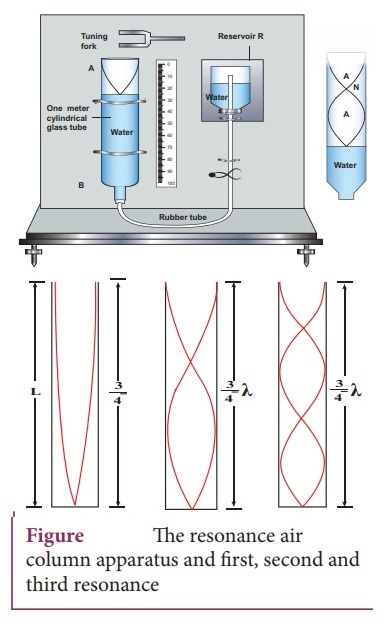● This cylindrical glass tube is mounted on a vertical stand with a scale attached to it.

● The tube is partially filled with water and the water level can be adjusted by raising or lowering the water in the reservoir R.

● The surface of the water will act as a closed end and other as the open end. Therefore, it behaves like a closed organ pipe, forming nodes at the surface of water and antinodes at the closed end.

● When a vibrating tuning fork is brought near the open end of the tube, longitudinal waves are formed inside the air column.

● These waves move downward and reach the surfaces of water and get reflected and produce standing waves.

● The length of the air column is varied by changing the water level until a loud sound is produced in the air column.

● At this particular length the frequency of waves in the air column resonates with the frequency of the tuning fork (natural frequency of the tuning fork).

● At resonance, the frequency of sound waves produced is equal to the frequency of the tuning fork.

● This will occur only when the length of air column is proportional to (1/4) th of the wavelength of the sound waves produced.

Let the first resonance occur at length L1, then 1/4 λ = L1

● But since the antinodes are not exactly formed at the open end, we have to include a correction, called end correction e, by assuming that the antinode is formed at some small distance above the open end.

The first resonance is 1/4 λ = L1 + e, e - end correction.

● Now-the length of the air column is increased to get the second resonance. Let L2 be the length at which the second resonance occurs.

Again taking end correction into account, 3/4 λ = L2 + e

● In order to avoid end correction, the difference between equation gives

3/4 λ − 1/4 λ = (L2 + e) − (L1 + e)

1/2 λ = L2 L1 = Δ L

λ = 2 ΔL

16. What is meant by Doppler effect?.

Discuss the following cases

(1) Source in motion and Observer at rest

(a) Source moves towards observer

(b) Source moves away from the observer

(2) Observer in motion and Source at rest.

(a) Observer moves towards Source

(b) Observer resides away from the Source

(3) Both are in motion

(a) Source and Observer approach each other

(b) Source and Observer resides from each other

(c) Source chases Observer

(d) Observer chases Source

Doppler Effect:

When the source and the observer are in relative motion with respect to each other and to the medium in which sound propagates, the frequency of the sound wave observed is different from the frequency of the source. This phenomenon is called Doppler Effect.

1) Source in motion and Observer at rest.

(a) Source moves towards the observer:

● Suppose a source S moves to the right with a velocity vs and let the frequency of the sound waves produced by the source be fs. The velocity of sound in a medium is v.

● The compression are (sound wave front) produced by the source S at three successive instants of time.

● When S is at position x1 the compression is at C1.

● When S is at position x2, the compression is at C2 and similarly for x3 and C3.

● Assume that if C1 reaches the observer's position A then at that instant C2 reaches the point B and C3 reaches the point C.

● The distance between compressions C2 and C3 is shorter than distance between C1 and C2.

● This means the wavelength decreases when the source S moves towards the observer O (since sound travels longitudinally and wavelength is the distance between two consecutive compressions).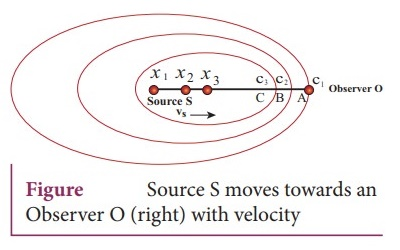● Frequency is inversely related to wavelength and therefore, frequency increases.

● Let λ be the wavelength of the source S as measured by the observer when S is at position x1 and λ' be wavelength of the source observed by the observer when S moves to position x2.

● The change in wavelength is Δλ = λ − λ' = vst, where t is the time taken by the source to travel between x1 and x2. Therefore, λ' = λ - vst , But t = λ / v

● On substituting equation t = λ / v in equation λ' = λ − vst, we get λ' = λ ( 1 – [vs / v])

● Since frequency is inversely proportional to wavelength, we have f ' = vs/λ' and f = vs / λ

Hence, f ' = f / ( 1 – [vs / v]), since vs / v << 1 we use the binomial expansion and retaining only first order in vs / v , we get   f ' = f ( 1 – [vs / v]) v(b) Source moves away from the observer:

● The velocity of the source is opposite in direction when compared to case (a), therefore, changing the sign of the velocity of the source in the above case i.e, by substituting (vs → -vs) in equation λ' = λ − vst,

we get f ' = f ( 1 – [vs / v])

● Using binomial expansion again, we get, f ' = f ( 1 – [vs / v])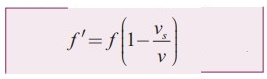2) Observer in motion and Source at rest.

(a) Observer moves towards Source● Let us assume that the observer O moves towards the source S with velocity vo .

● The source S is at rest and the velocity of sound waves produced by the source is v. Both vo and v are in opposite direction.

● Their relative velocity is vr = v + vo. The wavelength of the sound wave is λ = v / f , which means the frequency observed by the observer O is f ' = vr / λ . Then f ' = vr / λ = ([v + vo] / v) f = f (1 + [vo / v])(b) Observer recedes away from the Source

● If the observer O is moving away (receding away) from the source S, then velocity v0 and v moves in the same direction.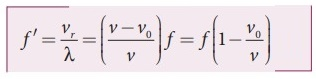● Therefore, their relative velocity is vr = v – v0. Hence, the frequency observed by the observer O is f ' = vr / λ . Then f ' = vr / λ = ([vv0] / v) f = f (1 – [v0 / v])

3) Both are in motion

(a) Source and observer approach each other

● Let Vs and v0 be the respective velocities of source and observer approaching each other.

● Let us have a dummy (behaving as observer or source) in between the source and observer. Since the dummy is at rest, the dummy (observer) observes the apparent frequency due to approaching source as given in equation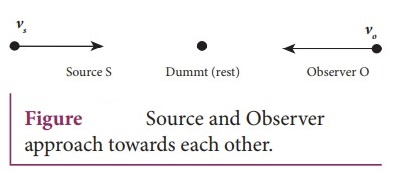● At that instant of time, the true observer approaches the dummy from the other side.

● Since the source (true source) comes in a direction opposite to true observer, the dummy (source) is treated as stationary source for the true observer at that instant.

● Hence, apparent frequency when the true observer approaches the stationary source (dummy source),

From equation f ' = vr / λ = ([v + v0] / v) f = f (1 + [v0 / v]) is

f ' = fd (1 + [v0 / v]) fd = f ' (1 + [v0 / v]) ……….(2)

● Since this is true for any arbitrary time, therefore, comparing equation (1) and equation (2), we get● Hence, the apparent frequency as seen by the observer is f ' = ([v + v0] / [vvs] ) f………… (3)

(b) Source and observer resides from each other

● Here, we can derive the result as in the previous case.

● The velocity of the source and the observer each point in opposite directions with respect to the case in (a) and hence, we substitute (vs → -vs ) and (v0 → -v0 ) in equation (3), and therefore, the apparent frequency observed by the observer when the source and observer recede from each other is

f ' = ([v - v0] / [v + vs] ) f      ………… (4)(c) Source chases observer

● Only the observer's velocity is oppositely directed when compared to case (a). Therefore, substituting (v0 → -v0) in equation .(3),

We get

f ' = ( [v - v0] / [vvs] ) f      …………(5)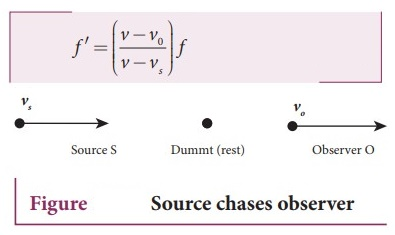(d) Observer chases source

● Only the source velocity is oppositely directed when compared to case (a). Therefore, substituting vs → -vs in equation (3),

We get f ' = ( [v + v0] / [v + vs] ) fTags : Waves | Physics , 11th Physics : UNIT 11 : Waves
Study Material, Lecturing Notes, Assignment, Reference, Wiki description explanation, brief detail
11th Physics : UNIT 11 : Waves : Long Questions and Answer | Waves | Physics